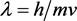﻿ 基于相对论的粒子波函数与实验分析

# 基于相对论的粒子波函数与实验分析Particle Wave Function and Experimental Analysis Based on Relativity Theory

Abstract: The Schrödinger equation uses the Planck constant as a characteristic quantity of the quantum mechanical system and is not easily solved in practice. The purpose of this paper is to establish a particle wave function equation based on the theory of relativity, which can be directly used to calculate particle experimental data and describe the physical reality of particle motion. This paper expounds the wave-particle duality of particle, introduces the particle’s rest mass constant into Einstein’s mass-energy equation of moving particles, introduces the particles moving mass into Newtonian kinetic energy equation, and the relativistic momentum operator, energy operatorand Hamiltonian operatorare proposed, and the Schrödinger equation is modified to be the relativistic particle wave function equation. In this paper, photon double-slit shooting experiments show that photons fluctuating in the lattice of air molecules, change their refractive index or reflectivity through the double-slit asymmetric energy field, and test proves that the position vector in the relativistic particle wave function equation is the particle fluctuating path vector. Through experimental data of hydrogen atom radiation, the electron wave function and probability wave of hydrogen atom are tested, and that the statistical explanation based onis in line with the physical reality of electron motion of hydrogen atoms is proved. The relativistic particle wave function will play an important role in the development and application of quantum physics.

1. 引言

1900年普朗克提出能量常数h假设，1905年爱因斯坦将普朗克常数h作为光子的能量常数，1924年，德布罗意  提出物质波假设，将普朗克常数h引入粒子或物质波计算公式。德布罗意认为光子的动量 $p=h/\lambda$ ，光子的波长 $\lambda =h/p$ ，并推导出物质波波长，或 $\lambda =h/\sqrt{2mE}$ ，其中m、E为实物粒子的质量、能量；实物粒子的频率 $\nu =E/h$ ，角频率 $\omega =E/\hslash$ ，波矢 $k=p/\hslash$ ，其中 $\hslash =h/2\text{π}$。依据德布罗意假设，自由粒子平面波函数

$\psi \left(r,t\right)=A\mathrm{exp}\frac{i}{\hslash }\left(p\cdot r-Et\right),$ (1)

1926年，薛定谔     将德布罗意物质波引入波函数理论，提出基于经典理论的波函数方程，描述粒子的波动性、运动状态和量子化性质，揭示粒子运动的基本规律。如一般情况下粒子微分运动薛定谔方程

$i\hslash \frac{\partial }{\partial t}\psi \left(r,t\right)=\left[-\frac{{\hslash }^{2}}{2m}{\nabla }^{2}+V\left(r,t\right)\right]\psi \left(r,t\right),$ (2)

$\left[-\frac{{\hslash }^{2}}{2m}{\nabla }^{2}+V\left(r\right)\right]\psi \left(r\right)=E\psi \left(r\right),$ (3)

1926年，玻恩   对薛定谔波函数进行统计诠释，认为薛定谔波函数是粒子几率波函数，论述了薛定谔波函数的合理性。

2. 粒子波函数的相关概念

2.1. 基本粒子波长与外场能量密度波长

2.2. 基本粒子波动与外场势能波动

2.3. 基于相对论的粒子波长与动量

1900年，普朗克提出的能量常数h，实际上是电子的能量常数。普朗克常数h与电子静止质量 ${m}_{e}$、电子能量E、康普顿电子波长 ${\lambda }_{c}$ 之间的关系， $h={m}_{e}c{\lambda }_{c}$$E=hc/{\lambda }_{c}$${\lambda }_{c}=h/{m}_{e}c$。1905年，爱因斯坦提出质能关系式 $E=m{c}^{2}$ ，电子的静止质量转换成能量 ${m}_{e}{c}^{2}=hc/{\lambda }_{c}$ ，所以 ${\lambda }_{c}=h/{m}_{e}c$${m}_{e}=h/c{\lambda }_{c}$。康普顿电子波长 ${\lambda }_{c}$ 是计算电子静止质量的波长。因此，依据基本粒子的波长(如各种光子的波长)计算其静止质量是合理的。粒子的质量也是量子化的，用粒子静止质量常数 ${h}_{m}=\text{5}\text{.45025577174353}×{\text{10}}^{-65}\text{\hspace{0.17em}}\text{kg}\cdot {\text{m}}^{3}$  和粒子波长计算粒子静止质量

$m=\frac{1}{\frac{4}{3}\text{π}{\lambda }^{3}}{h}_{m},$ (4)

$m=\frac{1}{\frac{4}{3}\text{π}{\left(\alpha \lambda /2\text{π}\right)}^{3}}{h}_{m}=\frac{6{\text{π}}^{2}}{{\alpha }^{3}{\lambda }^{3}}{h}_{m},$ (5)

${v}_{e}=\alpha c/\sqrt{\frac{{\lambda }_{e}}{{\lambda }_{0}}},$ (6)

${\lambda }_{0}=\frac{{\lambda }_{e}}{{\left(\alpha c/{v}_{e}\right)}^{2}}$${\lambda }_{0}=k{\lambda }_{c},$ (7)

${\lambda }_{e}={\left(\alpha c/{v}_{e}\right)}^{2}{\lambda }_{0}.$ (8)

$E=\frac{hc}{{\lambda }_{e}}.$ (9)

${m}_{m}=m/\sqrt{1-\left({v}^{2}/{c}^{2}\right)}-m,$ (10)

$m+{m}_{m}=m/\sqrt{1-\left({v}^{2}/{c}^{2}\right)}.$ (11)

$E={m}_{m}{c}^{2}.$ (12)

$E=\left(\frac{1}{2}m+{m}_{m}\right){v}^{2},$ (13)

${m}_{m}{c}^{2}=r\left(\frac{1}{2}m+{m}_{m}\right){v}^{2}.$ (14)

$E=\frac{1}{2}\left(m+{m}_{m}\right){v}^{2},$ (15)

${m}_{m}{c}^{2}=r\frac{1}{2}\left(m+{m}_{m}\right){v}^{2}.$ (16)

$p=\left(m+{m}_{m}\right)v=\sqrt{\left(2m+{m}_{m}\right)E},$ (17)

3. 基于相对论的粒子波函数

3.1. 基于相对论的动量、能量与哈密顿量算符Table 1. Data table of particle rest mass, Particle wavelength, Particle wavelength radius, velocity, energy, momentum, Particle motion wavelength and de Broglie wavelength

$p=\frac{6{\text{π}}^{2}v{h}_{m}}{{\alpha }^{3}{\lambda }_{c}^{3}\sqrt{1-\left({v}^{2}/{c}^{2}\right)}},$ (18)

$k=\frac{4\text{π}}{\alpha \lambda }$ , $\omega =\frac{2\text{π}c}{\lambda }.$ (19)

$\beta =\frac{p}{k{h}_{m}}=\frac{3\text{π}v\lambda }{2{\alpha }^{2}{\lambda }_{c}^{3}\sqrt{1-\left({v}^{2}/{c}^{2}\right)}},$ (20)

$\stackrel{^}{p}\equiv i\beta {h}_{m}\nabla$ , $\stackrel{^}{E}=i\beta {h}_{m}\frac{\partial }{\partial t}$$\stackrel{^}{H}=-\frac{{\beta }^{2}{h}_{m}^{2}}{2m+{m}_{m}}{\nabla }^{2}+V\left(r,t\right),$ (21)

3.2. 基于相对论的粒子波函数方程

$\psi \left(r,t\right)=A\mathrm{exp}\frac{i}{\beta {h}_{m}}\left(p\cdot r-Et\right),$ (22)

${\psi }_{p}\left(r\right)=\frac{1}{{\left(2\text{π}\beta {h}_{m}\right)}^{3/2}}{\text{e}}^{\frac{i}{\beta {h}_{m}}\left(p\cdot r\right)},$ (23)

$i\beta {h}_{m}\frac{\partial }{\partial t}\psi \left(r,t\right)=\left[-\frac{{\beta }^{2}{h}_{m}^{2}}{2m+{m}_{m}}{\nabla }^{2}+V\left(r,t\right)\right]\psi \left(r,t\right),$ (24)

$\left[-\frac{{\beta }^{2}{h}_{m}^{2}}{2m+{m}_{m}}{\nabla }^{2}+V\left(r\right)\right]\psi \left(r\right)=E\psi \left(r\right),$ (25)

4. 杨氏双缝实验对波函数的检验

4.1. 双缝的折射与反射原理

4.2. 粒子的路径波与波函数方程

4.3. 量子态迭加原理

$\psi \left(r\right)=\frac{1}{{\left(2\text{π}\beta {h}_{m}\right)}^{3/2}}\int \phi \left(p\right){\text{e}}^{\frac{i}{\beta {h}_{m}}\left(p\cdot r\right)}{\text{d}}^{3}p,$ (26)

$\phi \left(p\right)=\frac{1}{{\left(2\text{π}\beta {h}_{m}\right)}^{3/2}}\int \phi \left(r\right){\text{e}}^{\frac{i}{\beta {h}_{m}}\left(p\cdot r\right)}{\text{d}}^{3}x,$ (27)

4.4. 粒子的几率波函数

5. 氢原子辐射实验对波函数的检验

5.1. 氢原子的吸收与辐射

5.2. 氢原子外层电子波函数方程

$V=abe\left(\frac{1}{{\lambda }_{0}}-\frac{1}{{\lambda }_{e}}\right),$ (28)

$V=\frac{{e}^{2}}{8\text{π}{\epsilon }_{0}{r}_{e}}\left(\sqrt{{\lambda }_{0}/{\lambda }_{e}}-\frac{1}{\sqrt{{\lambda }_{0}/{\lambda }_{e}}}\right),$ (29)

$\left[-\frac{{\beta }^{2}{h}_{m}^{2}}{2m+{m}_{m}}{\nabla }^{2}+V\left(r\right)\right]\psi =E\psi ,$ (30)

5.3. 氢原子外层电子几率波

6. 结论Table 2. Data sheet of Hydrogen atom Palmer line system steady-state wave function and electron momentum probabilistic wave function

NOTES

*通讯作者。

 de Broglie, L. (1925) Recherches sur la théorie des quanta (Researches on the Quantum Theory). Annales de Physique, 3, 22. https://tel.archives-ouvertes.fr/tel-00006807/document

 吴先金. 普朗克常数与光子静止质量常数统一实验分析[J]. 现代物理, 2016(6): 183-193.

 Erwin, S. (1926) Quantisierung als Eigenwertproblem. Annalen der Physik, 384, 273-376.
https://doi.org/10.1002/andp.19263840404

 Erwin, S. (1926) Quantisierung als Eigenwertproblem. Annalen der Physik, 384, 489-527.
https://doi.org/10.1002/andp.19263840602

 Erwin, S. (1926) Quantisierung als Eigenwertproblem. Annalen der Physik, 385, 437-490.
https://doi.org/10.1002/andp.19263851302

 Erwin, S. (1926) Quantisierung als Eigenwertproblem. Annalen der Physik, 386, 109-139.
https://doi.org/10.1002/andp.19263861802

 Born, M. (1926) Das Adiabatenprinzip in der Quantenmechanik. Zeitschrift für Physik, 40, 167-192.
https://doi.org/10.1007/BF01400360

 Born, M. and Fock, V. (1928) Beweis des Adiabatensatzes. Zeitschrift für Physik, 51, 165-180.
https://doi.org/10.1007/BF01343193

 Nakatsuji, H. (2005) General Method of Solving the Schroedinger Equation of Atoms and Molecules. Physical Review A, 72, Article ID: 062110.
https://doi.org/10.1103/PhysRevA.72.062110

 Hernando de Castro, A. and Vanicek, J. (2013) Imaginary-Time Nonuniform Mesh Method for Solving the Multidimensional Schröedinger Equation: Fermionization and Melting of Quantum Lennard-Jones Crystals. Physical Review A, 88, Article ID: 062107.

 Wallstrom, T.C. (1994) Inequivalence between the Schröedinger Equation and the Madelung Hydrodynamic Equations. Physical Review A, 3, 1613-1617.
https://doi.org/10.1103/PhysRevA.49.1613

 Ariel, G., Christian, J. and Kaertner, F.X. (2006) Numerical Solver of the Time-Dependent Schröedinger Equation with Coulomb Singularities. Physical Review A, 73, Article ID: 042505.

 Arevalo, E. (2009) Soliton Theory of Two-Dimensional Lattices: The Discrete Nonlinear Schröedinger Equation. Physical Review Letters, 102, Article ID: 224101.
https://doi.org/10.1103/PhysRevLett.102.224102

 Nakatsuji, H. (2004) Scaled Schröedinger Equation and the Exact Wave Function. Physical Review Letters, 93, Article ID: 30403.

 Guo, X.Y., Shi, S.Z., Xu, N., Xu, Z. and Zhuang, P.F. (2015) Magnetic Field Effect on Charmonium Formation in High Energy Nuclear Collisions. Physics Letters B, 751, 215-219.
https://doi.org/10.1016/j.physletb.2015.10.038

 Lahiri, M. (2011) Wave-Particle Duality and Polarization Properties of Light in Single-Photon Interference Experiments. Physical Review A, 83, Article ID: 045803.
https://doi.org/10.1103/PhysRevA.83.045803

 Corderoa, E., Nicolab, F. and Rodinoa, L. (2015) Wave Packet Analysis of Schrodinger Equations in Analytic Function Spaces. Advances in Mathematics, 278, 182-209.

 Saha, A., Talukdar, B. and Chatterjee, S. (2017) Bound-state momentum-space wave function of the quasi-one-dimensional hydrogen atom, Quantum Phys-ics.

 Akrivis, G.D. (1993) Finite Difference Discretization of the Cubic Schrödinger Equation. IAM Journal on Numerical Analysis, 13, 115-124.
https://doi.org/10.1093/imanum/13.1.115

 Pathria, D. and Morris, J.L. (1990) Pseudo-Spectral Solution of Nonlinear Schrödinger Equations. Journal of Computational Physics, 87, 108-125.
https://doi.org/10.1016/0021-9991(90)90228-S

 Akrivis, G.D., Dougalis, V.A. and Karakashian, O.A. (1991) On Fully Discrete Galerkin Methods of Second-Order Temporal Accuracy for the Nonlinear Schrödinger Equation. Numerische Mathematik, 59, 31-53.
https://doi.org/10.1007/BF01385769

 Wu, X.J. (2017) Atomic Model and Experimental Analysis Based on Particle Spin. Modern Physics, 7, 94-105.
https://doi.org/10.12677/MP.2017.74011

 Heisenberg, W. (1927) Über den anschaulichen Inhalt der quantentheoretischen Kinematik und Mechanik. Zeitschrift für Physik, 43, 172-198.
https://doi.org/10.1007/BF01397280

 Titchmarsh, E.C. (1948) Introduction to the Theory of Fourier Integrals. 2nd Edition, Chapter 5, Oxford Clarendon Press, Oxford.

Top